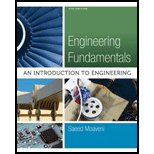Chapter 19, Problem 36P### Engineering Fundamentals: An Intro...

5th Edition
Saeed Moaveni
ISBN: 9781305084766

#### Solutions

Chapter
Section### Engineering Fundamentals: An Intro...

5th Edition
Saeed Moaveni
ISBN: 9781305084766
Textbook Problem

# For Problem 19.33, determine the probability (assuming normal distribution) that a bottle would be filled with more than 495 milliliters.

To determine

Calculate the probability for that a bottle would be filled with more than 495 milliliters.

Explanation

Given data:

The required values are shown in Table 1 with reference to the problem 19.33 in the textbook.

Formula used:

From equation 19.7 in the textbook, the formula to find mean for any sample is,

x¯=(xf)n (1)

Here,

x¯ is the mean,

x is the milliliters of water,

f is the frequency,

n is the total number of water bottles.

From equation 19.8 in the textbook, the formula to find standard deviation is,

s=(xx¯)2fn1 (2)

The formula to find the z score is,

z=xx¯s (3)

Here,

s is the standard deviation.

Calculation:

The calculation of terms in equation (1) is shown in Table 2

Here, the sum of the frequencies and the terms xf are,

f=n=151  and xf=75530

The total number of water bottles is the sum of the frequency, n=151.

Substitute 151 for n, and 75530 for xf in equation (1) to find x¯

x¯=75530151=500.2

The calculation of terms in equation (2) is shown in Table 3.

### Still sussing out bartleby?

Check out a sample textbook solution.

See a sample solution

#### The Solution to Your Study Problems

Bartleby provides explanations to thousands of textbook problems written by our experts, many with advanced degrees!

Get Started

## Additional Engineering Solutions

#### Find more solutions based on key concepts